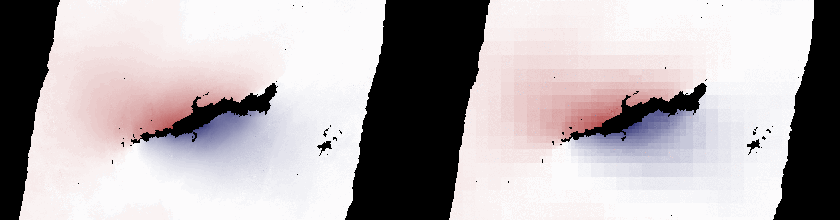The Quadtree reduces the amount of displacement data by sub-sampling the InSAR displacement map. For efficient forward modelling it is important to have reasonably sized dataset.Figure 1: Quadtree data reduction of an InSAR displacement scene. (Left) the origin full resolution ALOS displacement map, (right) the adaptive quadtree reduction.

The quadtree is made from hierarchically organized QuadNode, a slice through of the tree’s nodes is then called leaves.

Kite realises the quadtree concept from Jónsson et al. (2002) 1.

Note

All nodes of the Quadtree are built upon initialisation an instance.

The graphical user interface (GUI) spool offers an interactive parametrisation of the quadtree. Start the program, click on tab Quadtree. Detailed instruction can be found in spool’s tutorial.

Start spool and open a QuadTree container.
spool insar_displacement_scene.npz


The quadtree can also be parametrised by a python script. This example modifies the quadtree and saves the scene.

Tip

It is recommended to use the spool GUI for parametrisation of the quadtree and covariance.

import logging
from kite import Scene

logging.basicConfig(level=logging.DEBUG)

sc = Scene.import_data('test/data/20110214_20110401_ml4_sm.unw.geo_ig_dsc_ionnocorr.mat')

# For convenience we set an abbreviation to the quadtree

qt.epsilon = 0.024        # Variance threshold
qt.nan_allowed = 0.9      # Percentage of NaN values allowed per tile/leave

# Be careful here, if you scene is referenced in degree use decimal values!
qt.tile_size_max = 12000  # Maximum leave edge length in [m] or [deg]
qt.tile_size_min = 250    # Minimum leave edge length in [m] or [deg]

print(qt.reduction_rms)   # In units of [m] or [deg]
# >>> 0.234123152

for l in qt.leaves:
print l

# We save the scene in kite's format
sc.save('kite_scene')

# Or export the quadtree to CSV file
qt.export('/tmp/tree.csv')


## Plotting the quadtree with matplotlib¶

We can also use Matplotlib to plot the Quadtree’s current leaves.

import numpy as num

import matplotlib.pyplot as plt
from matplotlib import cm, colors

from kite import Scene

fig = plt.figure()
ax = fig.gca()

limit = num.abs(qt.leaf_medians).max()
color_map = cm.ScalarMappable(
norm=colors.Normalize(vmin=-limit, vmax=limit),
cmap=cm.get_cmap('RdBu'))

for rect, leaf in zip(qt.getMPLRectangles(), qt.leaves):
color = color_map.to_rgba(leaf.median)
rect.set_facecolor(color)